﻿
Reach Us+44-7482-875032
A Mathematical Model of Glucose-Insulin Interaction with Time Delay

# Journal of Applied & Computational MathematicsOpen Access

All submissions of the EM system will be redirected to Online Manuscript Submission System. Authors are requested to submit articles directly to Online Manuscript Submission System of respective journal.

# A Mathematical Model of Glucose-Insulin Interaction with Time Delay

Saber S1*, Bashier EBM2, Alzahrani SM3 and Noaman IA3
1Mathematics Department, Faculty of Science and Arts, Albaha University, Baljurashi, Saudi Arabia
2Department of Applied Mathematics, Faculty of Mathematical Sciences, University of Khartoum, Sudan
3Faculty of Arts and Science, Al-Baha University, Almandaq, Saudi Arabia
*Corresponding Author: Saber S, Mathematics Department, Faculty of Science and Arts, Albaha University, Baljurashi, Saudi Arabia, Tel: +966 17 727 4111, Email: [email protected]

Received Date: Sep 03, 2018 / Accepted Date: Sep 12, 2018 / Published Date: Sep 19, 2018

Keywords: Glucose-Insulin; Stability; Diabetes; Equilibrium point; Asymptotically

#### Introduction

Body cells need energy to function, and glucose is the main source of energy for the cells in the living organisms . Glucose comes from carbohydrate food after a meal intake. Therefore, the glucose level increases after food intake [2-4]. Here comes the role of the Insulin, which is a hormone that is produced by the Pancreas for the purpose of regulating the Glucose concentration in the blood [5-8]. The main function of the Insulin is to help the body cells to absorb the free blood glucose [9-11]. After its release by the Pancreas, the Insulin attaches with the cells and signals them to absorb the sugar from the blood stream [12-14].

Generally, two types of failure in controlling the level of sugar in the blood can occur. The first failure type happens when the Pancreas fails to produce enough amount of insulin . This failure type is referred to as juvenile diabetes. The second failure type happens when the body cells fail to respond to insulin in a proper way . The diabetes mellitus includes diabetes of types 1 and 2 [16,17].

According to the International Diabetes Federation (IDF), 387 million people have diabetes in 2014, and this number will rise to 592 million by 2035. The number of people with diabetes of type 2 is increasing in every country of the world. Moreover, Diabetes caused 4.9 million deaths in 2014; every seven seconds a person dies from diabetes (http://www.idf.org/diabetesatlas/update-2014).

The modeling of the glucose-insulin system has become an interesting topic, where, many mathematical models were developed to understand and predict the Glucose-Insulin dynamics [1,9,11,12,17]. Among the most widely used models to study diabetes dynamics, is the minimal model which is used in the interpretation of the intravenous glucose tolerance test . In this paper, we extend the work  by considering a time delay. We propose the following general model for the interaction of glucose and insulin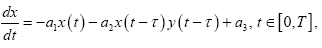(1)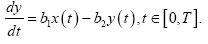with initial data:

x(θ)=ɸ(θ),θ<0, (2)

y(θ)=ψ(θ),θ<0 (3)

where x ≥ 0, y ≥ 0, x represents glucose concentration, y represents insulin concentration, a1 is the rate constant which represents insulinindependent glucose disappearance, a2 is the rate constant which represents insulin-dependent glucose disappearance, a3 is the glucose infusion rate b1 is the rate constant which represents insulin production due to glucose stimulation, b2 is the rate constant which represents insulin degradation and the time delay τ represents the time taken by Pancreas to respond to the feedback of the glucose level.

The rest of this paper is organized as follows. We discuss the qualitative analysis of the model behavior. The description of the numerical method for solving the proposed model is introduced. Then, we show the behavior of the model for different values of the time delay. Finally, these conclusions are disused.

#### Stability Analysis

In this we discuss the qualitative analysis of the model behavior. We shall now investigate the dynamics of the delay system eqn. (1). The steady state or equilibrium (fixed point) of the system eqn. (1) is one of which

x(t)=x(t−τ)=x(0)=x* ∀t,

y(t)=y(t−τ)=y(0)=y* ∀t

and as a consequence all the time derivatives vanish identically. Hence substituting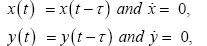in eqn. (1), it is easy to see that eqn. (1) has the equilibrium point (x*,y*) as the following: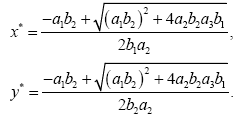The interior-equilibrium point E1=(x*,y*) exists unconditionally as x* and y* are always positive as all the parameters are considered positive.

To linearize the model about the equilibrium point E1 of model eqn. (1), let u(t)=x(t)−x*, v(t)=y(t)−y*. We then obtain the linearized model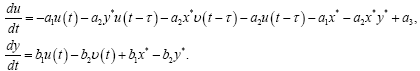(4)

After removing nonlinear terms, we obtain the linear vibrational system, by using equilibria conditions as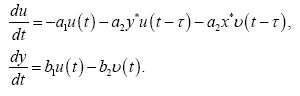(5)

From the linearized model we obtain the characteristic equation

Δ(λ,τ)=λ2+aλ+(bλ+c)e−λτ+d=0, (6)

where a=a1+b1, b=a2y*, c=a2b1x*+a2b2y* and d=a1b2.

For τ=0, the characteristic eqn. (6) becomes

Δ(λ,0)=λ2+(a+b)λ +c+d= 0, (7)

Now, sum of the roots=−(a+b) and product of the roots=c+d. Thus, we can say that both the roots of eqn. (7) are real and negative or complex conjugate with negative real parts if and only if

a+b>0 and c+d>0. (8)

Hence, in the absence of time delay, the equilibrium point E1 is locally asymptotically stable if and only if both conditions a+b>0 and c+d>0 hold simultaneously.

Following ; The interior-equilibrium point E1 is locally asymptotically stable when τ=0 if

(b1−a2x*)2<4b2(a1+a2y*). (9)

Now for τ≠ 0, if λ=iω(ω>0) is a root for the characteristic eqn. (6), then ω should satisfy the following equations

ω b sin ω τ+ccosω τ=ω2−d,

ω b cos ω τ – c sin ω τ=-aω, (10)

Which implies that+

ω4+(a2−b2−2d)ω2+d2−c2=0. (11)

Then we obtain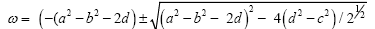From the eqn.(11), it follows that if

a2−b2−2d>0 and d2−c2>0 (12)

then eqn. (10) does not have any real solutions. From eqn. (11), we see that there is a unique positive solution w02 if

c2 −d2<0 (13)

If d2−c2>0, b2 −a2 −2d>0, and

(b2−a2−2d)2>4(d2−c2) (14)

hold, then there are two positive solutions ω±2 .

To find the necessary and sufficient conditions for nonexistence of delay induced instability, we now use the following theorem.

Theorem 1.1

A set of necessary and sufficient conditions for E1 to be asymptotically stable for all τ ≥ 0 is the following.

1. The real parts of all the roots of Δ(λ,0)=0 are negative .

2. For all real m and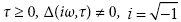Theorem 1.2

If conditions eqn.(8) and eqn.(12) and Theorem 1.1 are satisfied, then the equilibrium E1 is asymptotically stable for all τ<τ0 and unstable for τ>τ0. Furthermore, as τ increase through τ0, E1 bifurcates into small amplitude periodic solutions, where τ00n as n=0.

Proof: For τ=0, E1 is asymptotically stable if condition eqn. (8) holds. Hence, by Butler’s lemmma, E1 remains stable for τ<τ0. We now have to show that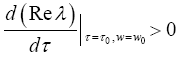This will signify that there exists at least one eigenvalue with positive real part for τ>τ0. Moreover, the conditions of Hopf bifurcation  are then satisfied yielding the required periodic solution.

Substituting w02 into eqn.(9) and solving for τ, we get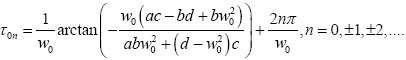(15)

Substituting ω±2 into eqn.(9) and solving for τ, we obtain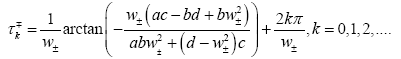(16)

Differentiating eqn. (6) w.r.t. τ, we obtain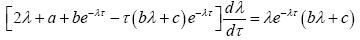(17)

thus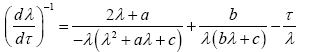(18)

and by using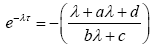we obtain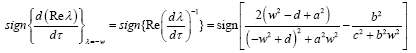(19)

It follows that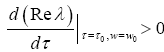.

Thus, the transversability condition holds, and hence, Hopf bifurcation occurs at τ=τ0. This completes the proof.

Theorem 1.3

Let τk ± be defined in eqn.(16). If in eqns. (8) and (14) are satisfied, then the equilibrium point E1 is stable when and unstable when, for some positive integer m. Thus, there are bifurcations at the equilibrium point E1 when τ=τk±, k=0,1,2,….

Proof: If conditions in eqn. (8) and (14) are satisfied, then to prove the theorem we need only to verify the transversability conditions [6,7].From eqn. (19), it follows that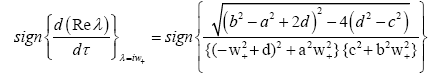Thus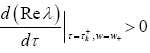Again,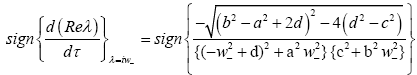Thus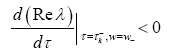Thus, the transferability conditions are satisfied.

#### Description of the Numerical Method

In this we follow the work [3,4] to design a positivity preserving numerical method for solving model.

Let N be any positive integer, and h=T/N. Let , tj = j = h for j=0,...,N. Let xj ≈ x(tj) and yj ≈ y(tj). Then, model eqn. (1) can be approximated by the non-standard finite difference formula: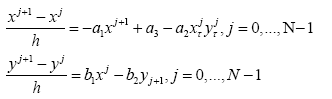from which we obtain the two relations: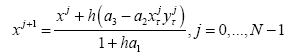(20)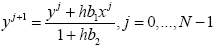(21)

where, xjτ approximates x(tj−τ) and yτj approximates y(tj−τ).

Let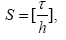then τ ≈ S·h. Now, x(tj −τ) can be approximated by x(tj−S) for j ≥ S and y(tj −τ) can be approximated by y(tj−S) for j ≥ S.

Then,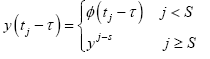(22)

and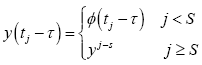(23)

#### Numerical Simulations

In this, section we give some numerical simulations supporting our theoretical predictions. This present some examples and numerical simulations to verify our theoretical results proved in the previous using matlab program.

We consider the system: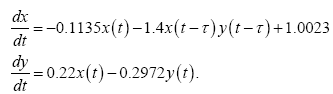There is a positive equilibrium (x*,y*)=(0.9302,0.6886).

Case I: For τ=0. In this case, the numerical simulation (Figures 1 and 2) shows that both the glucose and insulin converge in finite time to their equilibrium values x*=0.9302 and y*=0.6886, respectively.

Also in eqn. (9), i.e., the interior-equilibrium point E1 is locally asymptotically stable when τ=0 since

(b1−a2x*)2=0.9022<4b2(a1+a2y*)=1.4259.

Case II: For τ≠ 0, by Theorem 1.2, there is a critical value τ0=1.2738. The computer simulations (Figures 1-4), show that E1 is asymptotically stable when τ=1: 0738<τ0=1.2738. When τ passes through the critical value τ0=1.2738, E1 loses its stability and a Hopf bifurcation occurs, i.e., a family of periodic solutions bifurcate from E1. When τ>τ0=1.2738, E1 is unstable (Figures 5 and 6).

#### Conclusion

Delay differential equations are an interesting form of differential equations, with many different applications, particularly in the biological and medical worlds. In this paper, a mathematical model has been proposed and analyzed to study the dynamics of glucose and insulin in the human body. Numerical simulations are carried out to demonstrate the results obtained. Appropriately determining the range of the delay based on physiology and clinical data is important in theoretical study. All the numerical results and graphs presented in the project were in agreement with those presented in the relevant corresponding papers. Our results reveal the conditions on the parameters so that the periodic solution exist surrounding the interior equilibrium. It shows that τ0 is a critical value for the parameter τ. Furthermore, the direction of Hopf bifurcation and the stability of bifurcated periodic solutions are investigated. From the above results, we conclude that the model is physiologically consistent and may be a useful tool for further research on diabetes.

#### References

Citation: Saber S, Bashier EBM, Alzahrani SM, Noaman IA (2018) A Mathematical Model of Glucose-Insulin Interaction with Time Delay. J Appl Computat Math 7: 416. DOI: 10.4172/2168-9679.1000416

Copyright: © 2018 Saber S, et al. This is an open-access article distributed under the terms of the Creative Commons Attribution License, which permits unrestricted use, distribution, and reproduction in any medium, provided the original author and source are credited.

Select your language of interest to view the total content in your interested language

##### Recommended Journals
Viewmore
###### Article Usage
• Total views: 696
• [From(publication date): 0-0 - Aug 18, 2019]
• Breakdown by view type
• HTML page views: 611
• PDF downloads: 85

## Post your commentCan't read the image? click here to refresh
###### Peer Reviewed Journals

Make the best use of Scientific Research and information from our 700 + peer reviewed, Open Access Journals

International Conferences 2019-20

Meet Inspiring Speakers and Experts at our 3000+ Global Annual Meetings

Top